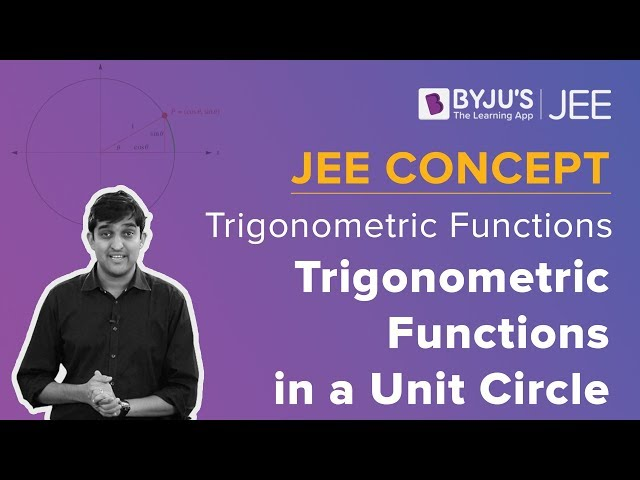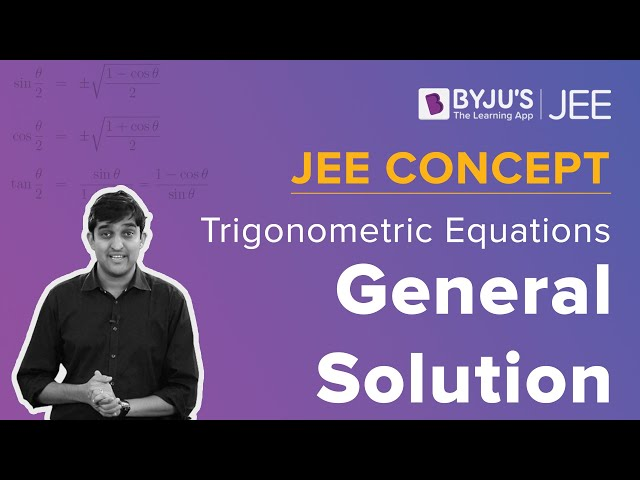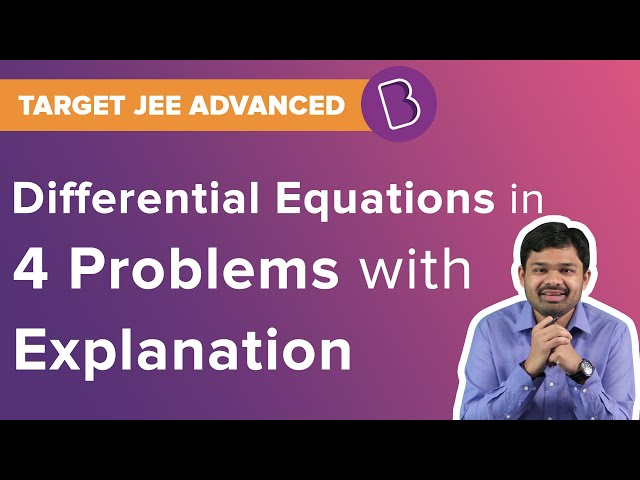Win up to 100% scholarship on Aakash BYJU'S JEE/NEET courses with ABNAT Win up to 100% scholarship on Aakash BYJU'S JEE/NEET courses with ABNAT

# JEE Main 2020 Maths Paper With Solutions Jan 7 Shift 2

JEE Main 2020 solved shift 2 Maths paper solutions are given here. PDF format of these solutions can be easily downloaded from our website. Learning these question papers help to understand the question pattern, marking scheme, and the difficulty level of questions from each chapter. Practising these solutions help students to improve their problem-solving skills and thus they can be stress-free and more confident during the exam.
January 7 Shift 2 – Maths
1. If 3x+4y =12√2 is a tangent to the ellipse (x2/a2) + (y2/9) = 1 for some a∈R then the distance between the foci of the ellipse is :
a. 2√5
b. 2√7
c. 2√2
d. 4

3x+4y =12√2 is a tangent to the ellipse (x2/a2) + (y2/9) = 1

Equation of tangent to ellipse (x2/a2) + (y2/9) = 1 is y = mx + √(a2 m2+ 9)

Now, 3x + 4y = 12√2 ⇒ y = -(3/4)x + 3√2

m = -3/4

And √(a2 m2+ 9) = 3√2

(a2 (-3/4)2+ 9) = 18

a2 (9/16) = 9

a2 = 16

a = 4

e = √(1-b2/a2)

e = √(1-9/16)

= √7/4

Distance between foci is 2ae = 2 × 4 × √7/4

= 2√7

2. Let A, B, C and D be four non-empty sets. The Contrapositive statement of “If A ⊆ B and B ⊆ D

then A ⊆ C ” is :
a. If A ⊆ C, then B ⊂ A or D ⊂ B
b. If A ⊈ C, then A ⊆ B and B ⊆ D
c. If A ⊈ C, then A ⊈ B and B ⊆ D
d. If A ⊈ C, then A ⊈ B or B ⊈ D

Given statements: A ⊆ B and B ⊆ D

Let A ⊆ B be p

B ⊆ D be q

A ⊆ C be r

Modified statement: (p

$$\begin{array}{l}\wedge\end{array}$$
𝑞) ⇒ r

Contrapositive: ~r ⇒ ~ (p

$$\begin{array}{l}\wedge\end{array}$$
𝑞)

~r ⇒ (~p

$$\begin{array}{l}\wedge\end{array}$$
~ q)

A ⊈ C, then A ⊈ B or B ⊈ D

3. The coefficient of x7 in the expression (1 + x)10 + x(1 + x)9 + x2(1 + x)8 + ⋯ +x10 is :
a. 420
b. 330
c. 210
d. 120

Coefficient of x7 in (1+x)10+ x(1+x)9+ x2(1+x)8+⋯ +x10

Applying sum of terms of G.P =

$$\begin{array}{l}\frac{(1+x)^{10}(1- (\frac{x}{1+x})^{11})}{(1-\frac{x}{1+x})}\end{array}$$

= (1 + x)11 − x11

Coefficient of x711C7 = 330

4. In a workshop, there are five machines and the probability of any one of them to be out of service on a day is ¼ . If the probability that at most two machines will be out of service on the same day is (3/4)3 k, then k is equal to:
a. 17/2
b. 4
c. 17/4
d. 17/8

P(Machine being faulty) = p = (1/4)

q = ¾

P(at most two machines being faulty)=P(zero machine being faulty)+P(one machine being

faulty)+P(two machines being faulty)

= 5C0 p0q5 + 5C1 p1q4 + 5C2 p2q3

= q5 + 5pq4 + 10p2q3

= (3/4)5 + 5×(1/4)×(3/4)4 + 10(1/4)2×(3/4)3

= (3/4)3[(9/16) + (15/16) + (10/16)]

= (3/4)3× (34/16)

= (3/4)3× (17/8)

k = 17/8

5. The locus of mid points of the perpendiculars drawn from points on the line x = 2y to the line x = y is:
a. 2x − 3y = 0
b. 3x − 2y = 0
c. 5x − 7y = 0
d. 7x − 5y = 0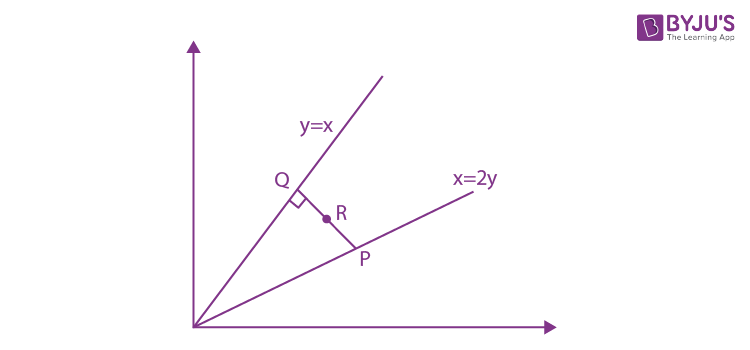Let R be the midpoint of PQ

PQ is perpendicular on line y = x

Equation of the line PQ can be written as y = −x + c

y = −x+c intersects y = x at Q: (c/2, c/2)

y = −x+c intersects x = 2y at P: (2c/3, c/3)

Midpoint R = (7c/12 , 5c/12)

Locus of R: x = 7c/12, y = 5c/12

5x – 7y = 0

6. The value of α for which
$$\begin{array}{l}4\alpha \int_{-1}^{2}e^{-\alpha \left | x \right |}dx = 5\end{array}$$
is:

a. loge 2
b. loge √2
c. loge (4/3)
d. loge (3/2)

$$\begin{array}{l}4\alpha \int_{-1}^{2}e^{-\alpha \left | x \right |}dx = 5\end{array}$$
$$\begin{array}{l}4\alpha \left [ \int_{-1}^{0}e^{-\alpha \left | x \right |}dx + \int_{0}^{2}e^{-\alpha \left | x \right |}dx \right ] = 5\end{array}$$
$$\begin{array}{l}4\alpha \left [ \int_{-1}^{0}e^{\alpha x}dx + \int_{0}^{2}e^{-\alpha x}dx \right ] = 5\end{array}$$
$$\begin{array}{l}4\alpha \left [ \left ( \frac{1-e^{-\alpha }}{\alpha } \right )+\left ( \frac{e^{-2\alpha }-1}{-\alpha } \right ) \right ] = 5\end{array}$$

⇒ 4[1 − e−2α − e−α + 1] = 5

Let e−α = t

⇒ 4t2 + 4t − 3 = 0

⇒ t = 1/2 = e−α

⇒ α = log𝑒 2

7. If the sum of the first 40 terms of the series, 3 + 4 + 8 + 9 + 13 + 14 + 18 + 19 + ….. is (102)m, then m is equal to :
a. 10
b. 25
c. 5
d. 20

S = 3 + 4 + 8 + 9 + 13 + 14 +…..40 terms

S = (3+4)+(8+9)+(13+14)+…..40 terms

S = 7 + 17 + 27 + 37 +….20 terms

This is an AP with a = 7, d = 10

S = (n/2)(2a+(n-1)d)

= (20/2)(14 + (19)10)

= (20/2)204

= 20 × 102

m = 20

8. If
$$\begin{array}{l}\frac{3+i\sin \theta }{4-i\cos \theta }, \theta \in [0,2\pi ]\end{array}$$
is a real number, then the argument of sin 𝜃 + 𝑖 cos 𝜃 is :

a. π- tan-1 (4/3)
b. – tan-1 (3/4)
c. π- tan-1 (4/3)
d. tan-1 (4/3)

Let

$$\begin{array}{l}z=\frac{3+i\sin \theta }{4-i\cos \theta }\times \frac{4+i\cos \theta }{4+i\cos \theta }\end{array}$$
$$\begin{array}{l}= \frac{12-\sin \theta \cos \theta +i(4\sin \theta +3\cos \theta )}{16+\cos ^{2}\theta }\end{array}$$

𝑧 is real.

4 sin 𝜃 + 3 cos 𝜃 = 0

⇒ tan 𝜃 = -3/4 [𝜃 lies in 2𝑛𝑑 quadrant]

arg (sin θ+ i cosθ ) =π + tan-1 (cos 𝜃 /sin 𝜃) =π – tan-1(4/3)

9. Let 𝐴 = [𝑎𝑖𝑗] and B = [𝑏𝑖𝑗] be two 3 × 3 real matrices such that 𝑏𝑖𝑗 = (3)(𝑖+𝑗−2)𝑎𝑗𝑖, where 𝑖, 𝑗 = 1, 2, 3. If the determinant of 𝐵 is 81, then the determinant of 𝐴 is :
a. 1/9
b. 1/81
c. 1/3
d. 3

𝑏𝑖 = (3)(𝑖+𝑗−2)𝑎𝑗𝑖

B =

$$\begin{array}{l}\begin{bmatrix} 3^{0}a_{11} & 3a_{21} &3^{2}a_{31} \\ 3a_{12}&3^{2} a_{22} & 3^{3}a_{32}\\ 3^{2}a_{13}& 3^{3}a_{23} & 3^{4}a_{33} \end{bmatrix}\end{array}$$
$$\begin{array}{l}\left | B \right | = \begin{vmatrix} 3^{0}a_{11} & 3a_{21} &3^{2}a_{31} \\ 3a_{12}&3^{2} a_{22} & 3^{3}a_{32}\\ 3^{2}a_{13}& 3^{3}a_{23} & 3^{4}a_{33} \end{vmatrix}\end{array}$$

Taking 32 common each from C3 and R3

$$\begin{array}{l}\left | B \right | = 81\begin{vmatrix} a_{11} & 3a_{21} &a_{31} \\ 3a_{12}&3^{2} a_{22} & 3a_{32}\\ a_{13}& 3a_{23} & a_{33} \end{vmatrix}\end{array}$$

Taking 3 common each from C2 and R2

$$\begin{array}{l}\left | B \right | = 81\times 9\begin{vmatrix} a_{11} & a_{21} &a_{31} \\ a_{12}& a_{22} & a_{32}\\ a_{13}& a_{23} & a_{33} \end{vmatrix}\end{array}$$

Given ǀBǀ = 81

81 = 81×9 ǀAǀ

ǀAǀ = 1/9

10. Let f(x) be a polynomial of degree 5 such that x = ± 1 are its critical points. If
$$\begin{array}{l}\lim_{x\to 0} \left (2+\frac{f(x)}{x^{3}} \right ) = 4\end{array}$$
then which one of the following is not true?

a. f(1) –4f(–1) = 4
b. x = 1 is a point of maxima and x = −1 is a point of minimum of f.
c. f is an odd function.
d. x = 1 is a point of minima and x = −1 is a point of maxima of f.

Given

$$\begin{array}{l}\lim_{x\to 0} \left (2+\frac{f(x)}{x^{3}} \right ) = 4\end{array}$$
$$\begin{array}{l}\lim_{x\to 0} \left (\frac{f(x)}{x^{3}} \right ) = 2\end{array}$$
$$\begin{array}{l}\lim_{x\to 0} \left (\frac{f(x)}{x^{3}} \right )\end{array}$$
limit exists and it is finite.

f(x) = ax5 + bx4 + cx3

$$\begin{array}{l}\lim_{x\to 0} (ax^{2} + bx +c) = 2\end{array}$$

c = 2

also f’(x) = 5ax4 + 4bx3 + 6x2

f’(1) = 5a + 4b + 6 = 0

f’(-1) = 5a – 4b + 6 = 0

b = 0 and a = -6/5

f(x) = -(6/5)x5 + 2x3

f(x) is odd

f’(x) = -6x4+ 6x2

f’’(x) = -24x3 +12x

(f’’(1) < 0)

(f’’(-1) > 0)

At x = -1 there is local minima and at x = 1 there is local maxima.

And f(1) -4f(-1) = 4

11. The number of ordered pairs (r, k) for which 6 ⋅ 35Cr = (k2 − 3) ⋅ 36Cr+1 , where k is an integer, is :
a. 4
b. 6
c. 2
d. 3

Using 36Cr+1 = (36/r+1)×35Cr

(36/r+1)×35Cr×(k2-3) = 35Cr×6

k2 – 3 = (r+1)/6

k2 = ((r+1)/6) +3

k∈I

r → Non-negative integer 0 ≤ r ≤ 35

r = 5 ⇒ k = ±2

r = 35 ⇒ k = ±3

No. of ordered pairs (r, k) = 4

12. Let a1, a2, a3, … be a G.P. such that a1 < 0, a1 + a2 = 4 and a3 + a4 = 16. If
$$\begin{array}{l}\sum_{i=1}^{9}a_{i} = 4\lambda\end{array}$$
then λ is equal to :

a. 171
b. 511/3
c. -171
d. -513

a1 + a2 = 4 ⇒ a + ar = 4 ⇒ a(1 + r) = 4

a3 + a4 = 16 ⇒ ar2 + ar3 = 16 ⇒ ar2(1 + r) = 16 ⇒ 4r2 = 16

⇒ r = ±2

If r = 2, a = 4/3

whch is not possible as a1 < 0

If r = −2, a = −4

$$\begin{array}{l}\sum_{i=1}^{9}a_{i}\end{array}$$
= a(r9-1)/(r-1)

= (-4)[(-2)9 -1]/-3

= 4/3)(-512-1)

= 4(-171)

λ = -171

13. Let a, b,c be three unit vectors such that
$$\begin{array}{l}\vec{a}+\vec{b}+\vec{c} = 0\end{array}$$
. If
$$\begin{array}{l}\lambda =\vec{a}.\vec{b}+\vec{b}.\vec{c}+\vec{c}.\vec{a}\end{array}$$
and

$$\begin{array}{l}\vec{d} =\vec{a}\times \vec{b}+\vec{b}\times \vec{c}+\vec{c}\times \vec{a}\end{array}$$
, then the ordered pair (λ,
$$\begin{array}{l}\vec{d}\end{array}$$
) is equal to:

a. (3/2, 3a×c)
b. (-3/2, 3c×b)
c. (-3/2, 3a×b)
d. (3/2, 3b×c)

Given a, b,c be three unit vectors.

a + b + c =0

= a.b + b.c + c.a

ǀa + b+cǀ2 = ǀ10ǀ2

ǀaǀ2 + ǀbǀ2 +ǀcǀ2 + 2(a.b + b.c + c.a) = 0

λ= -3/2

Also vector d = a×b + b×c + c×a

vector d = a×b + b×(-a-b) + (-a-b)×a

vector d = a×b + a×b + a×b

vector d = 3(a×b)

14. Let y = y(x) be the solution curve of the differential equation (y2-x)(dy/dx) = 1 satisfying y(0) = 1

This curve intersects the x − axis at a point whose abscissa is :

a. 2+e
b. 2
c. 2-e
d. -e

(y2– x)dy/dx = 1

(dx/dy) + x = y2

xey = ∫ y2eydy

x = y2 – 2y + 2 + ce-y

Given y(0) = 1

c = -e

Solution is x = y2 -2y + 2 – e-y+1

the value of x where the curve cuts the x axis will be at x = 2-e

15. If θ1 and θ2 be respectively the smallest and the largest values of θ in (0,2π) − {π} which satisfy the equation
$$\begin{array}{l}2\cot ^{2}\theta -\frac{5}{\sin \theta }+4 = 0\end{array}$$
then
$$\begin{array}{l}\int_{\theta _{1}}^{\theta _{2}}\cos ^{2}3\theta d\theta\end{array}$$
is equal to:

a. 2π/3
b. π/3
c. (π/3) + (1/6)
d. π/9

$$\begin{array}{l}2\cot ^{2}\theta -\frac{5}{\sin \theta }+4 = 0\end{array}$$
, θ∈[0,2π)

⇒ 2cosec2θ – 2 – 5cosec θ + 4 = 0

⇒2 cosec2θ – 4cosec θ – cosec θ + 2 = 0

⇒ cosec θ= 2 or ½ (not possible)

As θ ε[0,2π),

⇒ θ1 =π /6, θ2 = 5π/6

$$\begin{array}{l}\int_{\theta _{1}}^{\theta _{2}}cos^{2}3\theta d\theta = \int_{\frac{\Pi }{6}}^{\frac{5\Pi }{6}} \frac{(1+cos6\theta )}{2}d\theta\end{array}$$

= (1/2) [(5π/6)-(π/6)] + (sin 6θ/12) ǀ5π/6 π/6

= π/3

16. Let α and β are the roots of the equation x2 − x − 1 = 0. If pk = (α)k + (β)k, k ≥ 1 then which one of the following statements is not true?
a. (p1 + p2 + p3 + p4 + p5) = 26
b. p5 = 11
c. p5 = p2 ⋅ p3
d. p3 = p5 − p4

Given α, β are the roots of x2 − x − 1 = 0

⇒ α + β = 1 & αβ = −1

⇒ α2 = α + 1 & β2 = β + 1

pk = αk−2α2 + βk-2β2

pk = αk−2(α + 1) + βk−2(β + 1)

pk = α k−1 + β k−1 + α k−2 + β k−2

⇒ pk = p k−1 + p k−2

⇒ p3 = p2 + p1 = 4

p4 = p3 + p2 = 7

p5 = p4 + p3 = 11

∴ p5 ≠ p2 ⋅ p3 & p1 + p2 + p3 + p4 + p5 = 26

& p3 = p5 − p4

17. The area (in sq. units) of the region {(x, y)ϵR|4x2 ≤ y ≤ 8x + 12} is :
a. 125/3
b. 128/3
c. 124/3
d. 127/3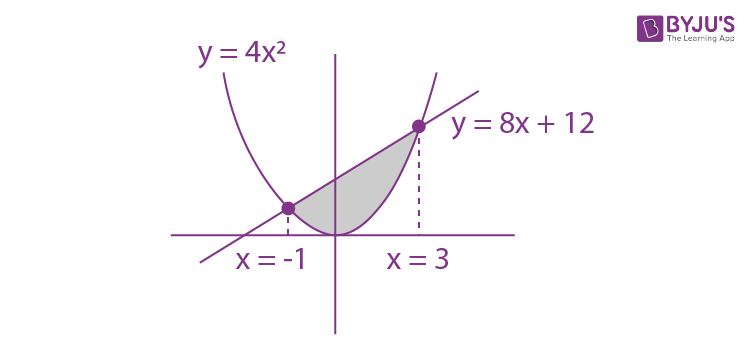For point of intersection,

4x2 = 8x + 12

⇒ x2 − 2x − 3 = 0

⇒ x = 3, −1

Area bounded is given by

$$\begin{array}{l}A = \int_{-1}^{3}(8x+12-4x^{2})dx\end{array}$$
$$\begin{array}{l}A = \left [ \frac{8x^{2}}{2}+12x – \frac{4x^{3}}{3} \right ]^{3}_{-1}\end{array}$$

A = (36 + 36 -36) – (4-12 + 4/3)

= 44 – (4/3)

= 128/3

18. The value of c in Lagrange’s mean value theorem for the function f(x) = x3 − 4x2 + 8x + 11, where x ∈ [0,1] is
a. (4 – √7)/3
b. 2/3
c. (√7 – 2)/3
d. (4 – √5)/3

LMVT is applicable on f(x) in [0,1], therefore it is continuous and differentiable in [0,1]

Now, f(0) = 11, f(1) = 16

f (x) = 3x2 − 8x + 8

f’(c) = (f(1) – f(0))/(1-0)

= (16 – 11)/1

⇒ 3c2 − 8c + 8 = 5

⇒ 3𝑐2 − 8𝑐 + 3 = 0

c = (8±2√7)/6 = (4±√7)/3

As c ∈ (0,1)

We get c = (4 – √7)/3

19. Let y = y(x) be a function of x satisfying y√(1 − x2)= k − x√(1 − y2) where k is a constant and y(1/2) = -1/4. Then dy/dx at x = ½ is equal to:
a. -√5/2
b. √5/2
c. -√5/4
d. 2/√5

y√(1 − x2)= k − x√(1 − y2)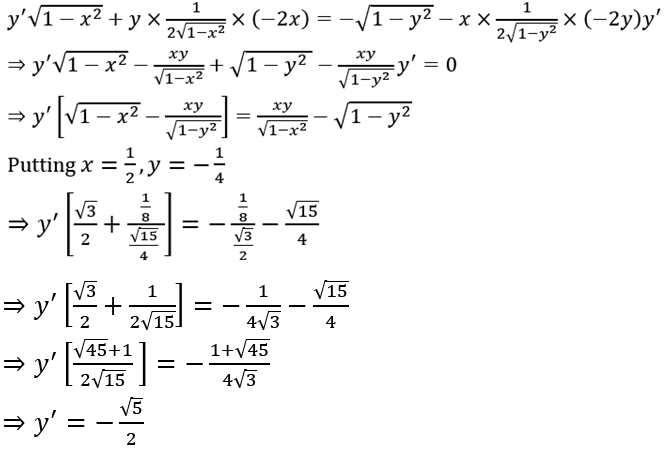20. Let the tangents drawn from the origin to the circle, x2 + y2 − 8x − 4y + 16 = 0 touch it at the points 𝐴 and 𝐵. The (𝐴𝐵)2 is equal to
a. 32/5
b. 64/5
c. 52/5
d. 56/5

x2 + y2 − 8x − 4y + 16 = 0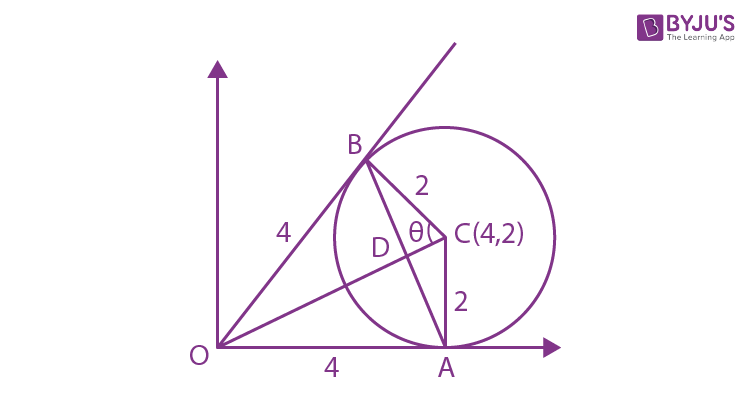(x − 4)2 + (y − 2)2 = 4 ⇒ Centre (4, 2) , radius = 2

OA = 4 = OB

In ∆OBC

tan θ= 4/2 = 2 ⇒ sin θ = 2/√5

In ∆BDC

sin θ= BD/2 ⇒ BD = 4/√5

Length of chord of contact (𝐴𝐵) = 8/√5

Alternative

(𝑙) length of tangent = 4 and (𝑟) radius = 2

⇒Length of chord of contact = 2lr /(√(l2+ r2)

Square of length of chord of contact = 64/5

21. If system of linear equations

x + y + z = 6

x + 2y + 3z = 10

3x + 2y + 𝜆z = 𝜇

has more than two solutions, then μ – λ2 is equal to

The system of equations has more than 2 solutions

∴ D = D3 = 0

$$\begin{array}{l}\begin{vmatrix} 1 & 1 &1 \\ 1 & 2 &3 \\ 3& 2 & \lambda \end{vmatrix} = 0\end{array}$$

2 λ− 6 −λ + 9 + 2 − 6 = 0

λ= 1

$$\begin{array}{l}\begin{vmatrix} 1 & 1 &6 \\ 1 & 2 &10 \\ 3& 2 & \mu \end{vmatrix} = 0\end{array}$$

⇒ μ = 14

So, μ − λ2 = 13

22. If the foot of perpendicular drawn from the point (1,0,3) on a line passing through (α, 7,1) is (5/3, 7/3, 17/3) then α is equal to:

Given points (1, 0, 3) and Q (5/3, 7/3, 17/3)

Direction ratios of line L : (α-5/3, 7-7/3, 1-17/3)

= ((3α-5)/3, 14/3, -14/3)

Direction ratios of line PQ : (-2/3, -7/3, -8/3)

As line L is perpendicular to PQ

((3α-5)/3)(-2/3) + (14/3)( -7/3) + (-14/3)(-8/3) = 0

-6α + 10 – 98 + 112 = 0

6α = 24

α= 4

23. If the function 𝑓 defined on (-1/3, 1/3) by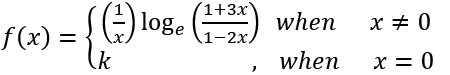is continuous, then k is equal to

As f(x) is continous

$$\begin{array}{l}\lim_{x\to 0 }f(x)= f(0) = k\end{array}$$
$$\begin{array}{l}\lim_{x\to 0 }(\frac{1}{x})log_{e}\frac{(1+3x)}{(1-2x)}= k\end{array}$$
$$\begin{array}{l}\lim_{x\to 0 }\frac{3log(1+3x)}{3x}+\lim_{x\to 0 }\frac{(-2)log(1-2x)}{-2x}= k\end{array}$$

3+2 = k

k = 5

24. If the mean and variance of eight numbers 3, 7, 9, 12, 13, 20, x and y be 10 and 25 respectively then xy is equal to

Mean = 10

(64 + x + y)/8 = 10

x + y = 80 – 64 = 16

Variance =

$$\begin{array}{l}\frac{\sum x_{i}^{2}}{n}- (\bar{x})^{2}\end{array}$$

25 = ((32 + 72 + 92 + 122 + 132 + 202 + x2 + y2 )/8 )- 100

1000 = 852 + x2 + y2

(x + y)2 – 2xy = 148

256 – 2xy = 148

2xy = 108

So xy = 54

25. Let 𝑋 = {𝑛 ∈ 𝑵: 1 ≤ 𝑛≤ 50}. If A = {𝑛 ∈ 𝑋: 𝑛 is a multiple of 2} and B = {𝑛 ∈ 𝑋: 𝑛 is a multiple of 7}, then the number of elements in the smallest subset of 𝑋 containing both A and B is .

A = {x: x is multiple of 2} = {2,4,6,8, … }

B = {x: x is multiple of 7} = {7,14,21, … }

X = {x ∶ 1 ≤ x ≤ 50, x ∈N}

Smallest subset of X which contains elements of both A and B is a set with multiples of 2 or 7 less than 50.

P = {x: x is a multiple of 2 less than or equal to 50}

Q = {x: x is a multiple of 7 less than or equal to 50}

n(P ∪ Q) = n(P) + n(Q) − n(P ∩ Q)

= 25 + 7 − 3

= 29